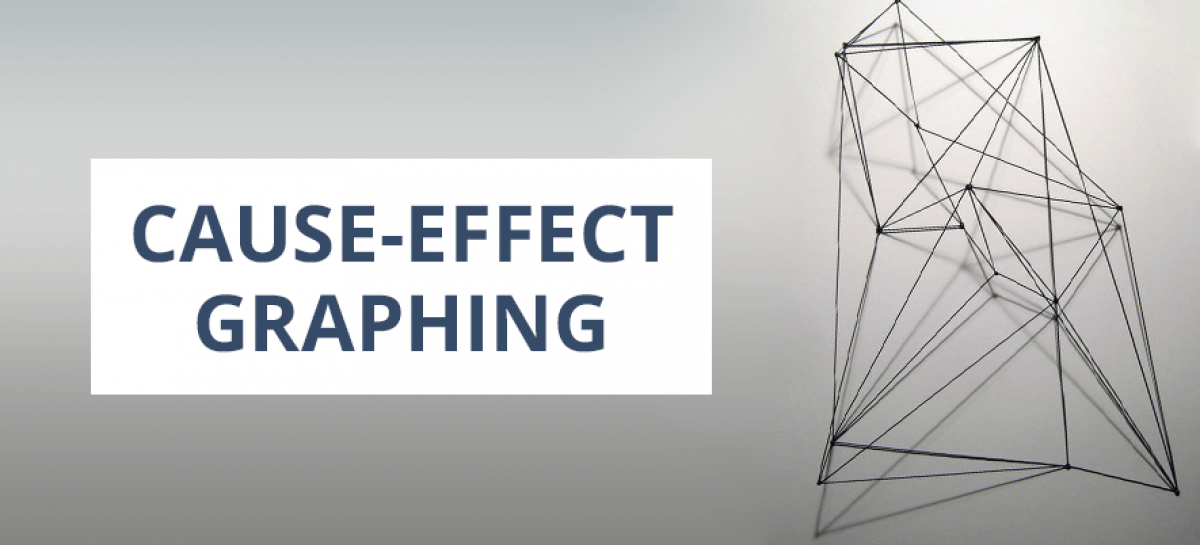# Black Box Test Techniques. Cause-Effect GraphingSeptember 13 10:00 2011

## Cause-effect Graphing

Design
Test cases should be designed to exert principles, which characterize the interrelation between the inputs and outputs of the component, where every principle corresponds to a singular possible combination of inputs to the component that have been expressed as Booleans.

For every test case these should be clarified:

• Boolean state for each effect
• Boolean state (true or false) for every reason

Analysis
Cause-Effect Graphing uses such model of the logical interrelations between causes and effects for the component. Every cause is expressed as a condition, which can be true of false on an input, or combination of inputs to the component. Every effect is expressed as a Boolean expression representing results, or a combination of results, for the component having occurred.

The model is characteristically represented as a Boolean graph relating the derived input and output Boolean expressions using the Boolean operators:

• AND
• OR
• NAND
• NOR
• NOT

## State Transition Testing

Design
Test cases should be designed to exert transitions between states. A test case may exercise any number of transitions.

For every test case these should be clarified:

• the starting state of the component;
• the input to the component;
• the expected outputs from the component;
• the expected final state.

For every expected transition within a test case these should be clarified:

• the beginning state;
• the event which is the reason of transition to the next state;
• the expected event which is the reason of transition;
• the expected next state.

Test cases are designed to exert valid transitions between states.

Analysis
State transition testing uses such model of the states the component may occupy, the transitions between states, the events which is the reason of transition, and the acts which can be the outcome of such transitions.

This model should include states, events, transitions, acts and their interrelation. The states of this model should be disjoint, identifiable and limited in quantity. Events which are the reason of transitions between states, and transitions may return to the state from which they started. The reason of events will be the inputs to the component, and acts in the state transition model may be the reason of the outputs from the component. The model will characteristically be represented as a state transition diagram, state table, or a state transition model.

## Boundary Value Analysis

Design
Test cases should be designed to exert values both on and next to the boundaries of the divisions. For each identified boundary 3 test cases should be produced corresponding to values on the boundary and an incremental distance either side of it. This rising distance is described as the smallest essential value for the data kind under consideration.

Normally test case should include:

• the partition boundaries exerted;
• the input to the component;
• the expected result of the test case.

Test cases are designed to exert valid boundary values, and invalid input boundary values. As well test cases can be designed to verify that invalid output boundary values can’t be induced.

Analysis
Boundary Value Analysis uses a model of the component that partitions the input values and output values of the component into a quantity of ordered sets with identifiable boundaries. Input and output values are derived from the specification of the component’s behaviour.

Such model should include bounded divisions of ordered input and output values. Each division should include a set or line of values, chosen in such a way that all the values can rationally be expected to be treated by the component in the equal way. Valid and invalid values are partitioned in this way. A partition’s boundaries are generally defined by the values of the boundaries between divisions, nevertheless where partitions are disunite the min and max values in the range which makes up the partition are used. The boundaries of valid and invalid partitions are considered.

## Equivalence Partitioning

Design
Test cases should be designed to exert divisions. A test case may exert any quantity of divisions. A test case shall comprise the following:

• the divisions exerted;
• the input to the component;
• the expected result of the test case.

Test cases are designed to exert divisions of valid values, and invalid input values. As well test cases can be designed to verify that invalid output values cannot be induced.

## Analysis

Equivalent partition uses a model of the component that partitions the output and input values of the component. The input and output values are derived from the specification of the component’s behaviour.

Such model should include divisions of input and output values. Each division should hold a set or line of values, elected in such way that all the values can rationally be expected to be treated by the component in the equal way. Both valid and invalid values are partitioned in this way.

## About Article Author

view more articlesNataliia Vasylyna

View More Articles

## Related Articles

view more articles
• http://www.centre4testing.com Richard

Hi Anastasia, just a note to say how much I have enjoyed reading your posts on a whole range of test matters.